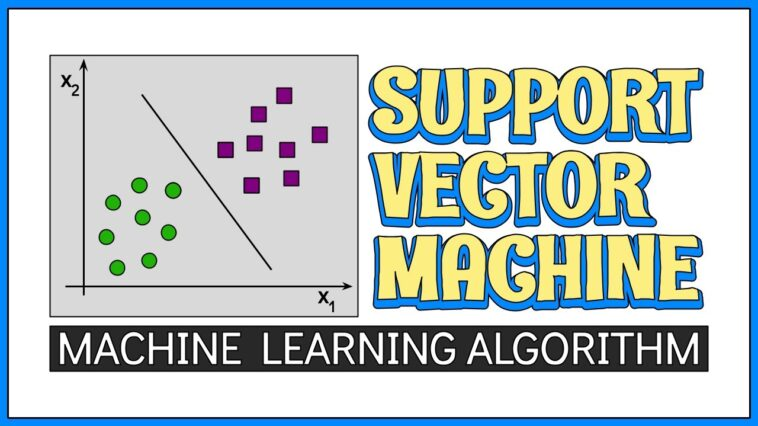in

# SVM vs. Other Machine Learning Algorithms: Which One to Choose – 2023 GuideMachine learning has revolutionized the field of artificial intelligence, and it has become an essential tool for businesses to improve their decision-making and enhance their operations. However, choosing the right machine learning algorithm can be challenging, given the plethora of options available. In this guide, we will compare SVM (Support Vector Machines) with other popular machine learning algorithms and help you make an informed decision.

## What is SVM?

Support Vector Machines is a popular machine learning algorithm used for classification and regression analysis. It is a binary classifier that separates data points into two categories using a hyperplane. SVM aims to find the optimal hyperplane that maximizes the margin between the two classes, ensuring that the classifier can generalize well to new data.

## SVM vs. Other Machine Learning Algorithms

Now let’s compare SVM with some other popular machine learning algorithms:

### Decision Trees

Decision Trees are a popular classification algorithm that uses a tree-like model to classify instances based on their features. They are easy to understand and interpret, and they can handle both categorical and continuous data. However, decision trees tend to overfit the data, which can reduce their generalization performance.

### Random Forests

Random Forests are an ensemble learning method that combines multiple decision trees to improve classification performance. They are more robust than decision trees as they reduce the risk of overfitting. However, they can be computationally expensive and require a large amount of data to train.

### K-Nearest Neighbors

K-Nearest Neighbors is a simple and intuitive classification algorithm that classifies instances based on their nearest neighbors in the feature space. It is easy to implement and can handle both categorical and continuous data. However, it can be sensitive to outliers and requires a large amount of data to perform well.

### Naive Bayes

Naive Bayes is a probabilistic classification algorithm that uses Bayes’ theorem to classify instances based on their conditional probabilities. It is simple to implement and can handle high-dimensional data. However, it assumes that the features are independent, which may not hold true in some cases.

## Why Choose SVM?

Now that we have compared SVM with other popular machine-learning algorithms, let’s discuss why you should choose SVM for your machine-learning tasks:

### SVM can handle high-dimensional data efficiently

SVM can handle high-dimensional data efficiently as it only requires a subset of the data points to compute the optimal hyperplane. This makes SVM particularly useful for tasks such as image classification, where the data may have a large number of features.

### SVM can handle non-linear data

SVM can handle non-linear data by using kernel functions to map the data to a higher-dimensional feature space where a linear hyperplane can separate the data. This makes SVM particularly useful for tasks such as text classification, where the data may not be linearly separable.

## Conclusion